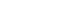Loading... Please wait...

# BST 322 Quiz 3 (National University)

Price:
\$14.99

Click the button below to add the BST 322 Quiz 3 (National University) to your wish list.

SHARE

## Product Description

1. In inferential statistics, the objective is to determine how probable it is that:
2. In medical statistics, we general set the level of significance at:
3. If you wanted to compare the effect of 3 separate kinds of interventions (3 separate groups of one independent variable -the intervention type) on a single dependent variable, the appropriate test would be:
4. A nurse researcher studied the effect of biofeedback on blood pressure. For this study, she measured blood pressure on subjects, taught them biofeedback techniques, then re-measured blood pressure on the same group of patients. Which t-test would be most appropriate for this design?
5. What does it mean if your test result is "statistically significant"?
6. When the alternative hypothesis states that the difference between two groups can only be in one direction, we call this a:
7. What is the probability of rolling an odd number on a fair die (singular of dice) in one roll?
8. If you wanted to test a set of patients' responses to a given therapy at 1, 3, and 6 months, you would use which statistical test?
9. The Central Limit Theorem states:
10. Nurse researchers hypothesized that the use of therapeutic touch would reduce anxiety levels in pre-op hip replacement patients. The null hypothesis would be:
11. A range of values estimated to have a high probably of containing the population value is called:
12. Level of significance is symbolized by:
13. The area of a sampling distribution beyond which the null hypothesis is rejected is known as the:
14. What is the probability of rolling first an odd number, then an even number with one fair die?
15. The hypothetical result of an infinite sample from a given population and calculating of some statistic from those infinite samples is a:
16. If a researcher rejects a null hypothesis when that hypothesis is actually true, he has committed:
17. The smaller the SEM,
18. Statistical tests that make assumptions about the underlying distributions are called:
19. If a researcher accepts a null hypothesis when that hypothesis is actually false, he has committed:
20. A study in which a set of patients are tested for pain level before and after being given a new pain medication would use which test?

## Product Reviews

This product hasn't received any reviews yet. Be the first to review this product!

• \$5.00
• \$14.99
• \$14.99
• \$14.99
• \$14.99

• \$5.00
• \$14.99
• \$14.99
• \$14.99
• \$14.99

## Related Products

• \$14.99• \$14.99• \$14.99• \$14.99• \$12.99